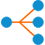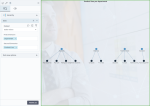# Hierarchy network chartHierarchy chart

A hierarchy network chart shows hierarchical relationships between entities. Entities represent two dimensions and are displayed as round nodes. Each node representing the first dimension is connected with the associated set of nodes representing the second dimension.

## When to use

Use a hierarchy network chart to visualize hierarchical relationships between entities (for example, between departments and product lines or between districts and the related schools).

## Data requirements

To build this chart, define the required and optional data fields as follows:

• First dimension – One dimension
• Second dimension – One dimension

Important: This widget can visualize up to 100 data rows.

## Use case

The following chart shows how product lines are hierarchically distributed across departments.Tip: The first dimension is always plotted above the second dimension. So, if you want to show a typical hierarchy where the top node is the parent of the lower nodes, you need to add the parent node (for example, a department) as the first dimension; otherwise, the chart will be plotted upside down.

## References

For details on how to customize your visualization, see Visualization settings.

For a whole list of visualizations, see the following topics: